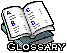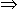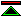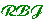..B

biconditional
a name used for the logical operator which yields a true proposition iff both its operands have the same truth value. So called because it is equivalent to the conjunction of two conditionals (implications), which is reflected by the usual symbol "", which consists of the conditional "" overlaid in two directions.
bind
tie or fasten tightly
(in logic)
the effect of various syntactic constructs (such as quantifiers or abstractions) which bind free occurrences of variables (of a certain name), within some expression, to a binding occurrence (of that same name) associated with a binder (e.g. a quantifier). A variable free in some expression, is no longer free in the larger expression formed by binding that variable. e.g. two free occurrences of the variable "x" in the expression "x=x" are bound when the universal closure is formed yielding "x. x=x", in which there are no longer any free occurrences of "x".
bivalent
A logic is bivalent if sentences in the logic can take either of just two truth values, usually named "true" and "false" or "T" and "F".
boolean
(after George Boole) often used as if it means "two-valued" or bivalent, but in fact allows any collection of values which conforms to certain algebraic laws (i.e. is a boolean algebra). A boolean propositional logic, though it admits interpretations in which propositional variables range over more than two values, has just the same set of theorems (the propositional tautologies) as a bivalent propositional logic.
boolean operator
An operator which takes boolean arguments and returns a boolean result.
bound
set bounds to, limit
(in logic)
to limit the scope of a variable, usually by enclosing that scope in some variable binding construction which identifies the variable thus bound.
bound variable
a variable whose scope has been restricted by some variable binding construct or declaration.©created 1999/9/16 modified 1999/10/6